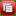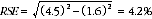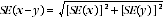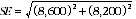6238.0 - Retirement and Retirement Intentions, Australia, Aug 2004 to Jun 2005
Previous ISSUE Released at 11:30 AM (CANBERRA TIME) 06/02/2006
 Page tools: .mffeedback,#pjs { display :none; } Enable Javascript to Print PagesPrint AllEmail NotificationRSSSearch this Product Explanatory Notes Glossary Abbreviations Appendix Data Quality (Technical Note) TECHNICAL NOTE DATA QUALITY INTRODUCTION 1 Since the estimates published in Retirement and Retirement Intentions, Australia, (cat. no. 6238.0) are based on information obtained from occupants of a sample of dwellings, they are subject to sampling variability. That is, they may differ from those estimates that would have been produced if all dwellings had been included in the survey. One measure of the likely difference is given by the standard error (SE), which indicates the extent to which an estimate might have varied by chance because only a sample of dwellings was included. There are about two chances in three (67%) that a sample estimate will differ by less than one SE from the number that would have been obtained if all dwellings had been included, and about 19 chances in 20 (95%) that the difference will be less than two SEs. Another measure of the likely difference is the relative standard error (RSE), which is obtained by expressing the SE as a percentage of the estimate. 2 In general, the size of the SE increases as the size of the estimate increases. Conversely, the RSE decreases as the size of the estimate increases. Very small estimates are thus subject to such high RSEs that their value for most practical purposes is unreliable. In the tables in this publication, only estimates with RSEs of 25% or less are considered reliable for most purposes. Estimates with RSEs greater than 25% but less than or equal to 50% are preceded by an asterisk (e.g. *3.4) to indicate they are subject to high SEs and should be used with caution. Estimates with RSEs of greater than 50%, preceded by a double asterisk (e.g. **0.3), are considered too unreliable for general use and should only be used to aggregate with other estimates to provide derived estimates with RSEs of less than 25%. CALCULATION OF STANDARD ERROR AND RELATIVE STANDARD ERROR 3 RSEs for all tables are provided. The RSEs have been derived using the group jack-knife method. SEs can be calculated using the estimates (counts or means) and the corresponding RSEs. 4 An example of the calculation of the SE from an RSE follows. Table 2.1 shows that the estimated number of females aged 45-49 who retired from the labour force aged less than 45 years is 48,700. The corresponding RSE table for 2.1 shows that the RSE for this estimate is 17.6%. The SE is: SE of estimate = (RSE / 100) x estimate = 0.176 x 48700 = 8,600 (rounded to the nearest 100) 5 Therefore, there are about two chances in three that the value that would have been produced if all dwellings had been included in the survey will fall within the range 40,100 to 57,300 and about 19 chances in 20 that the value will fall within the range 31,500 to 65,900. This example is illustrated in the diagram below.Proportions and percentages 6 Proportions and percentages formed from the ratio of two estimates are also subject to sampling errors. The size of the error depends on the accuracy of both the numerator and the denominator. A formula to approximate the RSE of a proportion is given below. This formula is only valid when x is a subset of y.7 Considering Table 2.1, of the 1,687,700 females who were retired from the labour force, 551,700 or 32.7% were aged less than 45 years at retirement. The RSE of 551,700 is 4.5% and the RSE for 1,687,700 is 1.6% (as shown in the corresponding RSE table for 2.1). Applying the above formula, the RSE for the proportion of females who did not prefer to work more hours is:8 Therefore, the SE for the proportion of females aged 45-49 who retired from the labour force aged less than 45 years is 1.4 percentage points (=(32.7/100)x 4.2). Therefore, there are about two chances in three that the proportion of females aged 45-49 who retired from the labour force aged less than 45 years is between 31.3% and 34.1%, and 19 chances in 20 that the proportion is within the range 29.9% to 35.5%. Sums or Differences between estimates 9 Published estimates may also be used to calculate the sum of, or difference between, two survey estimates (of numbers, means or percentages). Such estimates are also subject to sampling error. 10 The sampling error of the difference between two estimates depends on their SEs and the relationship (correlation) between them. An approximate SE of the difference between two estimates (x-y) may be calculated by the following formula:11 The sampling error of the sum of two estimates is calculated in a similar way. An approximate SE of the sum of two estimates (x + y) may be calculated by the following formula:12 An example follows. From paragraph 4 the estimated number of females aged 45-49 who retired from the labour force aged less than 45 years is 48,700 and the SE is 8,600. From Table 2.1, the estimate of females aged 50-54 who retired from the labour force aged less than 45 years old is 63,900 and the SE is 8,200. The estimate of females aged 45-54 who retired from the labour force aged less than 45 years is: 48,700 + 63,900 = 112,600 13 The SE of the estimate of females aged 45-54 who retired from the labour force aged less than 45 years is:= 11,900 (rounded to the nearest 100) 14 Therefore, there are about two chances in three that the value that would have been produced if all dwellings had been included in the survey will fall within the range 100,700 to 124,500 and about 19 chances in 20 that the value will fall within the range 88,800 to 136,400. 15 While these formulae will only be exact for sums of, or differences between, separate and uncorrelated characteristics or subpopulations, it is expected to provide a good approximation for all sums or differences likely to be of interest in this publication. Document Selection These documents will be presented in a new window.